# Multiple choice questions on heat transfer

## Multiple choice questions with one or more answers

Question 1. A Cylinder of radius R made of a material having thermal conductivity K is surrounded by a parrallopied having inner hole of radius R and outer dimension of 3 R each. Thermal conductivity of parrallopied is 2K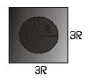The effective thermal conductivity of the system
a. $K[2 + \frac {\pi}{9}]$
b. $K[2 + \pi]$
c. $K[2 - \frac {\pi}{9}]$
d. $3 K$

$\frac {dQ_1}{dt}$ through Cylinder
$= K\pi R^2 (\frac {dT}{dL})$
$\frac {dQ_2}{dt}$ through parrallopied
$= 2K[9R^2- \pi R^2] (\frac {dT}{dL})$
if $K_{eq}$ is their thermal conductivity of system
$(\frac {dQ}{dt}) = K_{eq} 9R^2 (\frac {dT}{dL})$
then
$(\frac {dQ}{dt}) = (\frac {dQ_1}{dt})+(\frac {dQ_2}{dt})$
$K_{eq}9R^2 = K \pi R^2 +2 K [9R^2- \pi R^2]$
$K_{eq}= (\frac {K}{9})[18 - \pi ] = K[2 - \frac {\pi}{9}]$

Question 2. Three black bodies are such that higher intensity wavelengths are in the ratio
$\lambda_{m1} : \lambda_{m2} : \lambda_{m3} = 1 :(21)^{1/2}: (3)^{1/2}$
which of the these is true for the temperatures
a. $T_1 > T_3 > T_2$
b. $T_1 > T_2 > T_3$
c. $T_3 > T_2 > T_1$
d. $T_3 > T_1 > T_2$

$\lambda_{m1} = 1$
$\lambda_{m2} = (21)^{1/2}$
$\lambda_{m3}= (3)^{1/2}$
so $\lambda_{m2} > \lambda_{m3} > \lambda_{m1}$
so now as $\lambda_{m1}T= constant$
so $T_1 > T_3 > T_2$

Question 3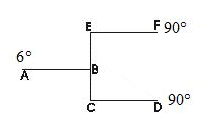All Rod are of equal length and equal area of cross-section. Find the temperature at point B
a. 45
b. 51.4
c. 50
d. 51

Let T be the temperature at B then total heat flow at B should be zero
$[\frac {KA(T-0)}{L}]+ [\frac {KA(T - 90)}{2L}] + [\frac {KA(T-90)}{2L}] = 0$
$T + \frac {(T - 90)}{2} + \frac {(T - 90)}{2} = 0$
$2T + (T-90) + (T - 90) = 0$
$2T + T + T = 180$
T = 45

Question 4
Three identical rods are connected between two container contained at Temp. $T_1$ and $T_2$ ($T_1 > T_2$ ) .There are three possible way to join the rod both the container as show below.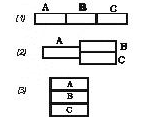Let $Q_1$, $Q_2$, $Q_3$ are the rate of heat flow for these three figure respectively
when of these is true.
a. $Q_3 > Q_2 > Q_1$
b. Thermal resistance is maximum in fig(a)
c. $Q_1 : Q_3 = 1 : 9$
d. All the above

$Q_1=[\frac {(T_1 - T_2)}{(\frac {L}{KA} + \frac {L}{KA} + \frac {L}{KA})]$
$=\frac {KA}{3L}(T_1 - T_2)$
$Q_3= (T_1 - T_2)( \frac {KA}{L}+ \frac {KA}{L}+\frac {KA}{L})$
$= (\frac {3KA}{L})(T_1 - T_2)$
$Q_2 = \frac {(T_1 - T_2)}{(R + R / 2)} = \frac {(T_1 - T_2)}{(\frac {L}{KA} + \frac {L}{2KA})}$
$=(T_1 - T_2)(\frac {2KA}{3L})$
Resistance in case (a)=$\frac {3L}{KA}$
Resistance in case (b)=$\frac {3L}{2KA}$
Resistance in case(c)=$\frac {L}{3KA}$
So resistance is maximum in case (a)
Also $Q_3 > Q_2 > Q_2$

A rod of thermal conductivity K and area A and length L is there. The initial temperature distribution along the rod is given by
$T = T_0 sin (\frac {\pi x }{ L})$
where $T_0$ is in ºC. Suppose both the end of the rod kept at 0ºC.

Question 5 which of these is the initial temperature distribution diagram

a.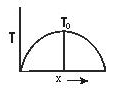b.c.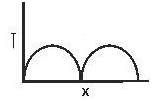d.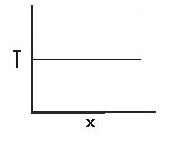$T = T_0 sin (\frac {\pi x }{ L})$

Question 6 What is the initial temperature gradient at the end of rods
a. $\frac {T_0 \pi}{L}$
b. $T_0 \pi$
c. $\frac {T_0 }{ L}$
d. $\frac {T_0}{\pi L}$

$T = T_0 sin (\frac {\pi x }{ L})$
$\frac {dT }{ dx} = T_0Cos ( \frac {\pi x }{ L}) \times \frac {\pi }{ L}$
$= (\frac {T_0 \pi}{L}) cos(\frac {\pi x }{ L})$
at x = 0
$(\frac {dT }{ dx})_{x=0} = \frac {T_0 \pi}{L}$
x=L
$(\frac {dT }{ dx})_{x=L} = - \frac {T_0 \pi}{L}$

Question 7 What is the initial heat current from the ends of the rods into the bodies making contact with its ends
a. $\frac {\pi K A T_0}{L}$
b. $\frac {KAT_0}{L}$
c. $\frac {3KAT_0}{L}$
d. $\frac {4KAT_0}{L}$

$Q=KA \frac {dT}{dx}$
As $\frac {dT}{dx}$ at ends is $\frac {T_0 \pi}{L}$
so $Q= \frac {\piKAT_0}{L}$

Question 8 What is the initial heat current at the center of rod
a. zero
b. $\frac {KAT_0}{L}$
c. $\frac {2KAT_0}{L}$
d. $\frac {2KAT_0}{3L}$

as $Q=KA \frac {dT}{dx}$
at center $\frac {dT}{dx} = 0$
so Q= zero

## Multiple choice questions with one or more answers

Question 9 Steady state temperature distribution along a rod is shown by which figure.
a.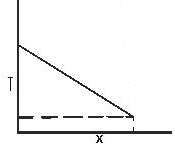b.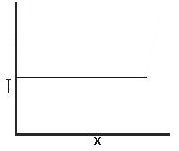c.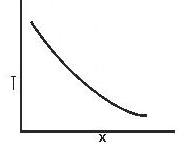d.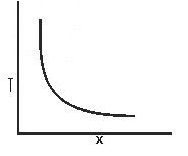Question 10 Which of these is true for a black body
a.(a = 1) , (e = 1)
c. frequency of radiation which is dominant is directly proportional to the temperature of the body
d. All of the above

Question 11
There are two bodies A & B they are having same surface and same mass. They are at temperature T at time t=0
Temperature of the surrounding is $T_0$
Given ($T > T_0$)
Let $a_1$ & $a_2$ are the absorptive power of the two surface and $a_1 > a_2$
which of these is true
a. Body A will cool faster than body B
b. Emissivity is greater for body B
c. Emissivity is greater for body A
d. Body B will cool faster than body A

$E_1 = \epsilon _1 \sigma A (T^4- T_0^4)$
for body B
$E_2 = \epsilon _2 \sigma A (T^4- T_0^4)$
Now it is given $a_1 > a_2$
so $\epsilon _1 > \epsilon _2$
so $E_1 > E_2$
So body A will cool faster than B
so a, c are correct

Two sphere A & B are there of same material
sphere A is hollow sphere
sphere B is solid sphere
Radius is same for both the sphere
They are at same temperature $T_0$

Question 12
A) dT/dt at t = 0 will be same for the sphere
(B) Emissive power of both the sphere will be same in the beginning

(a) A & B both are correct
(b) Only B is correct
(c) Only A is correct
(d) None of them is correct

There masses will be different
They emit same emissive power in the beginning as their surface area is same and they are of same material but temperature will depends on mass and heat emitted both. Since they are having different massed,dT/dt will not be same

Question 13 if the temperature difference between sphere and surrounding is T for both the sphere which one is true
a. A will cool faster for all values of T
b. A & B both will cool at same rate for all values of T
c. A & B will have equal temperature at any instant
d none of the above

From above explanation, it is evident that hollow sphere will cool faster as have less mass

Question 14 if the sphere A radius is doubled then what will be ratio of emissive power of sphere A & B in the beginning
a $E_1:E_2=4:1$
b. $E_1:E_2=1:4$
c. $E_1:E_2=2:1$
d. $E_1:E_2=1:2$

Emissive power depends on surface area so when radius is doubled ,surface area becomes four times

## Multiple choice questions with one answers

Question 15
A hot body whose temperature is T is kept in a room where temperature is $T_0$.The hot body takes time $t_1$ to cool from temperature T to $T_2$,time $t_2$ to cool from temperature $T_2$ to $T_3$,time $t_3$ to cool from temperature $T_3$ to $T_4$

Also $T > T_2 > T_3 > T_4 > T_0$
and $T - T_2=T_2 -T_3=T_3-T_4=a$
which one of the following is true
a. $t_1 > t_2 > t_3$
a. $t_3 > t_2 > t_1$
a. $t_1=t_2=t_3$
d. None of the above

$\frac {dT}{dt}=-k(T-T_0)$
So rate of cooling is depends on the temperature difference
It will be greatest in the beginning and will decrease subsequent
So for same temperature difference
$t_3 > t_2 > t_1$ (b) is correct

Question 16 if the temperature of the hot body is plotted against time which of the following curve will represent it
a.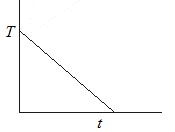b.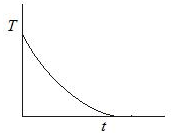c.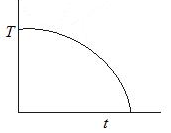d.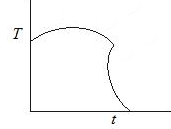Question 17 If the area is doubled and temperature is made halved what will be the ratio of power radiated
a $E_1:E_2=4=8:1$
b. $E_1:E_2=1:1$
c. $E_1:E_2=1:4$
d. $E_1:E_2=1:2$

$E_1= \sigma AT^4$
$E_2=\sigma (2A)(T/2)^4$
$E_1:E_2=4=8:1$

Question 18 Suppose A body behaves like black body. When the temperature of black-body increases, it is observed that the wavelength corresponding to maximum energy changes from $\lambda$ to $\frac {\lambda}{2}$. Find the ratio of emissive power of the body at respective
temperature
a $E_1:E_2=1:8$
b. $E_1:E_2=1:2$
c. $E_1:E_2=1:1$
d. $E_1:E_2=2:1$

$E_1=\sigma AT_1^4$
$E_2=\sigma AT_2^4$

Also according to Wien displacement law
$\lambda T=constant$
$\lambda T_1=\lambda (T_2/2)$

$T_1 : T_2=1:2$
So $E_1:E_2=1:8$

Question 19: Which of the following is the vm-T graph for a perfectly black-body. Here vm is the frequency at maximum emission of radiation and T is the absolute temperature of the body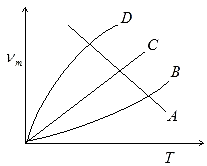λT=constant
as λ=1/vm

So vm=kT

So straight line

Question 20
A composite slab is prepared by pasting three slab of thickness $L_1$,$L_2$,$L_3$ and thermal conductivity $K_1$,$K_2$,$K_3$.The slab have equal cross-sectional area. Find the equivalent thermal conductivity
a.$\frac {K_1K_2K_3(L_1+L_2+L_3)}{K_2K_3L_1+K_1K_3L_2+K_1K_2L_3}$
b.$\frac {K_2K_3L_1+K_1K_3L_2+K_1K_2L_3}{K_1+K_2+K_3}$
c. $\frac {K_1+K_2+K_3}{L_1+L_2+L_3}$
d. $\frac {L_1+L_2+L_3}{K_1+K_2+K_3}$

$R=R_1+R_2+R_3$
$\frac {L_1+L_2+L_3}{KA}=\frac {L_1}{K_1A} + \frac {L_2}{K_2A}+ \frac {L_3}{K_3A}$

$K=\frac {K_1K_2K_3(L_1+L_2+L_3)}{K_2K_3L_1+K_1K_3L_2+K_1K_2L_3}$

Question 21
What increase in radiated power results when the temperature of a black-body is increased from 7 to 287 0 C
(a)4
(b) 8
(c) 16
(d) None of these

$\frac {P(560K)}{(280K)} = (\frac {560}{280})^4 =16$

Question 22
Absorbing power of the surface is .7. Transmitting power of the surface is .1
A total heat Q is incident on the surface. Find the heat reflected back?
(a)Q/6
(b)Q/4
(c) Q/2
(d) Q/5

We know that
a+r+t=1
.7+r+.1=1
r=.2
Now
r=Reflected energy/Total energy
So reflected energy=Q/5

Assertion and Reason
a) Statement I is true ,statement II is true ,statement II is correct explanation for statement I
b) Statement I is true ,statement II is true ,statement II is not a correct explanation for statement I
c) Statement I is true,Statement II is false
d) Statement I is False,Statement II is True
Question 23
STATEMENT 1:Thermal conduction is the heat transfer that occurs in the direction of temperature decrease
STATEMENT 2:Thermal conduction is solids occurs due to vibrational energy of the molecules about the equilibrium position

(b)

Question 24
STATEMENT 1: If a body is in thermal equilibrium with the surroundings then it will absorb and emit radiant energy at the same rate
STATEMENT 2:Stefan -Boltzmann law is true for both emmision and absorption of radiant energy

(a)

## Match the column

Question 25
Four rods of equal cross-section area A and L and thermal conductivity K are joined in four ways.Match the column I to column II
Column A
A. All rod are joined in series
B. All rod are joined in parallel
C. They are grouped in two pair having two rods each joined in parallel and both the pairs are joined are series
D.They are grouped in two pair .One pair having one rod and other pair having three rods joined in parallel.
Column B
P. Thermal Resistance of the system is 4L/KA
Q. Thermal Resistance of the system is L/4KA
R.Thermal Resistance of the system is 4L/3KA
S.Thermal Resistance of the system is L/KA

a.A->P,B->Q,C->S,D->R
b.A->P,B->Q,C->R,D->S
c.A->Q,B->P,C->S,D->R
d. A->P,B->S,C->Q,D->R

(a)

Question 26
A sphere, a cube and a thin circular plate, all of same material
and same mass are initially heated to same high temperature.
(a) Plate will cool fastest and cube the slowest
(b) Sphere will cool fastest and cube the slowest
(c) Plate will cool fastest and sphere the slowest
(d) Cube will cool fastest and plate the slowest

(c)

Question 27
A glass full of hot milk is poured on the table. It begins to cool gradually. Which of the following is correct?
(a) The rate of cooling is constant till milk attains the temperature of the surrounding.
(b) The temperature of milk falls off exponentially with time.
(c) While cooling, there is a flow of heat from milk to the surrounding as well as from surrounding to the milk but the net flow of heat is from milk to the surrounding and that is why it cools.
(d) All three phenomenon, conduction, convection and radiation are responsible for the loss of heat from milk to the surroundings

(b),(c),(d)